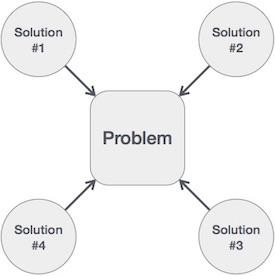# Python - Algorithm Design

Algorithm is a step-by-step procedure, which defines a set of instructions to be executed in a certain order to get the desired output. Algorithms are generally created independent of underlying languages, i.e. an algorithm can be implemented in more than one programming language.

From the data structure point of view, following are some important categories of algorithms −

• Search − Algorithm to search an item in a data structure.

• Sort − Algorithm to sort items in a certain order.

• Insert − Algorithm to insert item in a data structure.

• Update − Algorithm to update an existing item in a data structure.

• Delete − Algorithm to delete an existing item from a data structure.

## Characteristics of an Algorithm

Not all procedures can be called an algorithm. An algorithm should have the following characteristics −

• Unambiguous − Algorithm should be clear and unambiguous. Each of its steps (or phases), and their inputs/outputs should be clear and must lead to only one meaning.

• Input − An algorithm should have 0 or more well-defined inputs.

• Output − An algorithm should have 1 or more well-defined outputs, and should match the desired output.

• Finiteness − Algorithms must terminate after a finite number of steps.

• Feasibility − Should be feasible with the available resources.

• Independent − An algorithm should have step-by-step directions, which should be independent of any programming code.

## How to Write an Algorithm?

There are no well-defined standards for writing algorithms. Rather, it is problem and resource dependent. Algorithms are never written to support a particular programming code.

As we know that all programming languages share basic code constructs like loops (do, for, while), flow-control (if-else), etc. These common constructs can be used to write an algorithm.

We write algorithms in a step-by-step manner, but it is not always the case. Algorithm writing is a process and is executed after the problem domain is well-defined. That is, we should know the problem domain, for which we are designing a solution.

### Example

Let's try to learn algorithm-writing by using an example.

• Problem − Design an algorithm to add two numbers and display the result.

step 1 − START

step 2 − declare three integers a, b & c

step 3 − define values of a & b

step 4 − add values of a & b

step 5 − store output of step 4 to c

step 6 − print c

step 7 − STOP

Algorithms tell the programmers how to code the program. Alternatively, the algorithm can be written as −

step 2 − get values of a & b

step 3 − c ← a + b

step 4 − display c

step 5 − STOP

In design and analysis of algorithms, usually the second method is used to describe an algorithm. It makes it easy for the analyst to analyze the algorithm ignoring all unwanted definitions. He can observe what operations are being used and how the process is flowing.

Writing step numbers, is optional.

We design an algorithm to get a solution of a given problem. A problem can be solved in more than one ways.Hence, many solution algorithms can be derived for a given problem. The next step is to analyze those proposed solution algorithms and implement the best suitable solution.

## Useful Video Courses

Video

#### Python Online Training

187 Lectures 17.5 hours

Video

#### Python Essentials Online Training

55 Lectures 8 hours

Video

#### Learn Python Programming in 100 Easy Steps

136 Lectures 11 hours

Video

#### Python with Data Science

Best Seller

75 Lectures 13 hours

Video

#### Python 3 from scratch to become a developer in demand

Best Seller

70 Lectures 8.5 hours

Video

#### Python Data Science basics with Numpy, Pandas and Matplotlib

Most Popular

63 Lectures 6 hours